# Set theory

(diff) ← Older revision | Latest revision (diff) | Newer revision → (diff)
Jump to: navigation, search

naive

The study of the properties of sets (cf. Set), pre-eminently infinite, disregarding the properties of the elements in those sets. The idea of a set is one of the primitive mathematical ideas and can only be explained by means of examples. Thus, it is possible to speak of the set of people living on our planet at a given time, of the set of points of a given geometric figure and of the set of solutions of a given differential equation. A person living on the planet at the given time, a point of the given geometric figure, a solution of the given differential equation are elements of their respective sets. A setis regarded as given if a characteristic property of the elements of the set is given, that is, a property which all the elements of the set, and only they, possess. One of the fundamental ideas of set theory is that of membership of an element of a set. To denote that an objectbelongs to a setone writes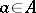(ifdoes not belong toone writes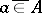or). (It can happen that no object has the characteristic property defining; it is then said thatis empty and one writes. For example, the set of real solutions of the equation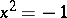is empty.) If each element of a setis at the same time an element of a set, thenis called a subset ofand one writes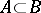. Ifand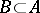simultaneously, thenandare said to be equal and one writes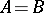. The union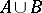of two setsandis the set comprising all the elements which belong to at least one of the setsor. The intersection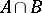ofandis the set comprising all the elements which belong to bothand. The operations of union and intersection are commutative, associative and mutually distributive. For example,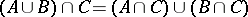. Often one considers only sets which are contained in some fixed set. Ifis a subset ofandis a property characterizing the elements of, then one writes. For example, ifis the set of all real numbers andis the subset of positive numbers, then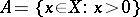. If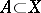, then the set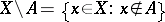is called the complement of the set(in). The operations of union, intersection and taking the complement are connected by the so-called de Morgan laws. For example,. The branch of set theory concerned with the study of operations on sets (not only finite, but also infinite operations) is called the algebra of sets. The algebra of sets, in its turn, is a special case of the theory of Boolean algebras (cf. Boolean algebra).

Set theory was created by the work of 19th century mathematicians, who posed the aim of a complete revision of the foundations of mathematical analysis. Already in the first papers in this area (B. Bolzano, P. du Bois-Reymond, R. Dedekind), which considered sets of numbers or functions, the question of a quantitative comparison of infinite sets was posed. Is infinity of a set purely a negative property, not admitting elaboration, or do there exist different levels of mathematical infinity; are there infinite sets of different quantitative strength, of different "power" ? The answer to this question was given by G. Cantor (1871–1883), who presented an almost modern exposition of the theory of cardinal and ordinal numbers (cf. Cardinal number; Ordinal number) and the theory of well-ordered sets (cf. Well-ordered set). The possibility of a comparative quantitative estimation of sets was based on the idea of a one-to-one correspondence (or bijection) between two sets. Associate to each element of a set, by any rule whatever, a definite element of a set. If each element ofturns out to be in correspondence to one and only one element of, then it is said that a one-to-one correspondence (or bijective mapping or bijection) has been established betweenand. There is a bijection between two finite sets if and only if both sets have the same number of elements. Generalizing this fact, Cantor defined quantitative equivalence, or equipotence, as the possibility of establishing a one-to-one correspondence between them. If a setis equipotent to a set, thenandhave the same cardinal number. The value of the notion of the power of a set is determined by the existence of non-equipotent infinite sets. For example, the set of all real numbers and the set of all natural numbers have different powers. The first has the power of the continuum, and the second is a countable set. In each infinite setthere is a proper subset ofequipotent to the whole of, whereas in a finite set such a proper part cannot be found. Therefore the presence of a proper part equipotent to the whole can be taken as the definition of an infinite set.

The merit of Cantor is not just in the solution of the problem of the power of a set, but in the decisive step he made of considering sets with elements of an arbitrary nature. To testify that this step to generality was difficult, there are firstly the various inconsistencies (cf. Antinomy), discoveries by various scholars at the beginning of the 20th century and leading to the creation of axiomatic set theory, and secondly, the emergence of various problems (for example, the continuum hypothesis) which turned out to be undecidable.

A subsequent contribution to set theory was made by F. Hausdorff, who, after developing the theory of totally ordered sets and applying set theory to topology, completed the foundations of the theory of topological spaces (cf. Topological space) (or, general topology). Later, the-operation, arising in the study of Borel sets, led to the creation of descriptive set theory. From a number of problems in combinatorial mathematics and graph theory, combinatorial set theory arose. Finally, the discoveries of K. Gödel and P. Cohen in axiomatic set theory significantly influenced the methods and development of set theory.